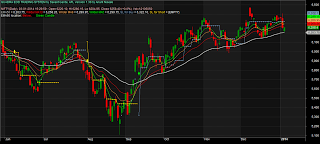Connect with us

### AmibrokerQuadra Trading System is best amibroker afl for the EOD based analysis .so be careful and calculated their value and show result get lot money.

## Similar Formulas

```/****************************/
/*                          */
/*       Version 1.30       */
/*     (11 August 2012)     */
/*                          */
/****************************/

SetChartOptions(0, chartShowDates | chartWrapTitle);

/**********************/
/*                    */
/* Define Wilder's MA */
/*                    */
/**********************/

function WilderMA(Field, Period)
{
X = Field;

for(i = 1; i < BarCount; i++)
{
X[i] = Field[i]/Period + X[i - 1] * (Period - 1)/Period;
}
return X;
}

/**********************/
/*                    */
/*     Define EMA     */
/*                    */
/**********************/

function MyEMA(Field, Period)
{
Y = Field;
for(i = 1; i < BarCount; i++)
{
Y[i] = (Field[i] - Y[i - 1]) * 2/(Period + 1) + Y[i - 1];
}
return Y;
}

/********************/
/*                  */
/*   Wilder's MAs   */
/*                  */
/********************/

A1 = WilderMA(C, 5);
A2 = WilderMA(C, 8);
A3 = WilderMA(C, 13);
A4 = MyEMA(C, 50);

/********************/
/*                  */
/*   Candle Color   */
/*                  */
/********************/

Green = C > O;
Red = C < O;

for(i = 1; i<BarCount; i++)
{
if(C[i] == O[i])
{
if(Green[i - 1] AND C[i] >= C[i - 1]) Green[i] = 1;
if(Red[i - 1] AND C[i] <= C[i - 1]) Red[i] = 1;
}
}

/********************/
/*                  */
/*    COMPUTE SL    */
/*                  */
/********************/

PrevHi = H;
PrevLo = L;
SLShort = Null;
GUp = GapUp();
GDn = GapDown();

for(i = 2; i < BarCount; i++)
{
PrevLo[i] = PrevLo[i - 1];

if(L[i - 2] >= L[i - 1] AND L[i - 1] <= L[i])
{
PrevLo[i] = L[i - 1];
}

PrevHi[i] = PrevHi[i - 1];
if(H[i - 2] <= H[i - 1] AND H[i - 1] >= H[i])
{
PrevHi[i] = H[i - 1];
}

}

/********************/
/*                  */
/*                  */
/********************/

Buy = (A1 > A2 AND A2 > A3);
Sell =(A1 < A2 AND A2 < A3);

for(i = 1; i <BarCount; i++)
{
SLShort[i] = Min(PrevHi[i], PrevHi[i - 1]) + 0.1;
if(GDn[i]) SLShort[i] = L[i - 1] + 0.1;
if(Sell[i]) break;
}
for(i = 1; i <BarCount; i++)
{
SLBuy[i] = Max(PrevLo[i], PrevLo[i - 1]) - 0.1;
if(GUp[i]) SLBuy[i] = H[i - 1] - 0.1;
}

for(i = 1; i < BarCount; i++)
{
if(GUp[i]) SLBuy[i] = H[i - 1] - 0.1;

if(Sell[i]) SLShort[i] = PrevHi[i - 1] + 0.1;
if(Sold[i]) SLShort[i] = Min(PrevHi[i - 1], PrevHi[i]) + 0.1;
if(GDn[i]) SLShort[i] = L[i - 1] + 0.1;
}
for(i = 1; i<BarCount; i++)
{
if(SLShort[i] > SLShort[i - 1]) SLShort[i] = SLShort[i - 1];
}

Marker1 = Buy * shapeUpArrow + Sell * shapeDownArrow;
Marker1Dist = IIf(Buy, 0.995 * L, 1.005 * H);
EMA_Position = IIf(A4 > A3 AND A4 > A2 AND A4 > A1, 1, IIf(A4 < A3 AND A4 < A2 AND A4 < A1, -1, 0));

/***********************/
/*                     */
/*   SHOW/HIDE Option  */
/*                     */
/***********************/

Arrows = ParamToggle("Show Arrows", "NO|YES");
ShowTSL= ParamToggle("Show TSL Line", "NO|YES");

_N(Title = EncodeColor(colorAqua) + "QUADRA EOD TRADING SYSTEM by Savant Garde; AFL Version 1.30 by Anant Navale\n\n"
+ EncodeColor(colorPink)  + StrFormat("{{NAME}}({{INTERVAL}}), {{DATE}} : {{OHLCX}}, Vol=%1.0f\n{{VALUES}}\n", V)
+ "EMA50 location: " + EncodeColor(colorBrightGreen) + WriteIf(EMA_Position > 0, "Above,     ", "")
+ EncodeColor(colorLightGrey) + WriteIf(EMA_Position == 0, "Inside,     ", "")
+ EncodeColor(colorRed) + WriteIf(EMA_Position < 0, "Below,     ", "")
+ EncodeColor(colorBrightGreen) + WriteIf(Green, " Green Candle", "")
+ EncodeColor(colorRed) + WriteIf(Red, " RedCandle\n\n", "\n\n")
+ EncodeColor(colorWhite) + WriteIf(Buy, "Buy Above " + (H + 0.1), "")
+ WriteIf(Sell, "Sell Below " + (L - 0.1), "")
+ WriteIf(Sell, "   StopLoss = " + SLShort, ""));

if(Status("action") == actionIndicator)
{
Plot(A4, "EMA50", colorLightGrey, styleLine | styleThick);
Plot(A3, "Wilder MA13", colorRed, styleLine);
Plot(A2, "Wilder MA8", colorLightOrange, styleLine);
Plot(A1, "Wilder MA5", colorBrightGreen, styleLine);

if(ShowTSL)
{
Plot(SLShort, "SL for Short", colorGold, styleDots);
}

Plot(C, "", IIf(Green, colorGreen, IIf(Red, colorRed, colorGrey50)), styleCandle);

if(Arrows)
{
PlotShapes(Marker1, MarkerColor, 0, MArker1Dist);
}
}

if(Status("action") == actionExplore)
{

SetOption("NoDefaultColumns", True);
}```

## Disclaimer

There is a substantial risk of loss associated with trading in Stock Markets. Losses can and
will occur. No responsibility for loss occurred to any person acting or refraining to act as a result of using the AFL written by their respective creators and published in this Blog for sharing of knowledge can be accepted by the Blog owner.

Click to comment

This site uses Akismet to reduce spam. Learn how your comment data is processed.